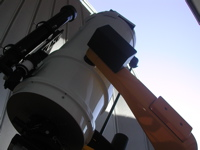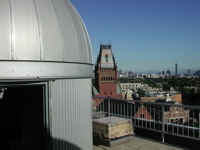# Astronomy Lab and Clay TelescopeVisual Observations of Double-Double

SPU-21 Fall 2015

printer version

The Double-Double, or Epsilon Lyrae, is an insteresting star system near the bright star Vega. It is a multiple star system but we'll focus on four stars that make up two inner binary star systems that orbit each other to make an outer binary system.

Procedure:

• Slew the telescope to Double-Double at coordinates RA= 18:44:48.57 DEC=+39:40:13.3 using TheSky software.
• First, look through the Finder Telescope. You should see two sets of double star systems. Can you see all four stars? We want to measure the small separation between inner pairs and then the larger separation between the outer pair.
• Next, look through the main eyepiece with the reticle and rotate the reticle so the line with the tick marks runs between the two stars in the outer pair (largest separation).
• Measure the angular separation: count the number of tick marks between the two stars (Each tick mark is ~5.7arcsec).
• Repeat this measurement for the Southern inner pair.

Analysis:

• We want to measure the orbital period of the inner and the outer binaries.
• First, calculate the angular separation of the inner and outer pairs of stars in arcseconds from your tick mark measurement. Use the conversion factor of 5.7 arcseconds/ tick mark. Combine your several measurements (in tick marks) to report the average and uncertainty (rms) of your measurement.
• Next, using the skinny triangle formula, θ (radians) = a(pc) / d(pc), where a is the separation of the stars in pc and d is the given distance (50pc) to the stars, determine the separation between the inner stars and the outer stars. Watch your units! You need to convert your angular separation from arcsec to radians to use it in the skinny triangle formula. (Note: 1 radian = 206,265 arcsec)
• Now, let's use Newton's version of Kepler's 3rd Law, P2(yrs) = a3 (AU) / Mstars, where P is the orbital period in years, a is the separation of the stars in AU and M is the mass of the stars in solar mass units (given Mstars = 2Msun). Again be careful of units! You will need to convert your separation, a, to AU. (Note: 1 pc =206,265 AU)
• Do this for both the inner and outer binaries.
• Make sure to hand in your quick sketch with your report.